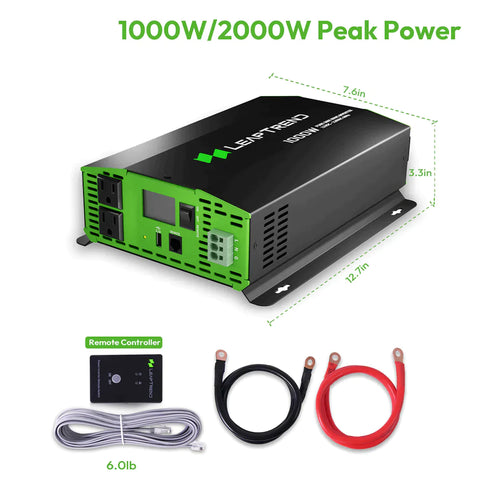# What Is The Minimum Battery For 1000W Inverter?

When choosing an inverter for home use, both the power rating and battery capacity are important considerations. In this article, we will explore the minimum battery capacity required for a 1000W inverter.

To determine the minimum battery capacity for a 1000W inverter, calculations need to be based on the load power and operating time. Typically, the specifications of the inverter provide information on its rated load power and operating time.

## Calculation Method for Required Battery Capacity of Inverter

Firstly, it is crucial to know the efficiency of the inverter. Generally, the working efficiency of an inverter ranges from 80% to 90%. This means that when the inverter is outputting 1000W, its input power will be between 1250W to 1111W.

At this time, the battery needs to provide a current of 1250/12 or 1111/12 (calculated according to a 12V inverter), which is approximately 104A or 92.5A. This means that a 100A battery can work for about one hour.

Secondly, the required working time needs to be determined based on individual needs and usage, such as 2 hours, 4 hours, or 8 hours.

Finally, we can use the following formula to calculate the minimum battery capacity required:

Minimum Battery Capacity (AH) = (Load Power of the Inverter (W) / Efficiency of the Inverter / 12) x Required Working Time (hours)## Required battery capacity for a 1000W inverter

For example, assuming an inverter with an efficiency of 90%, requiring a working time of 5 hours, and a load power of 1000W, we can use the following formula:

Minimum Battery Capacity (AH) = (1000W / 0.9 / 12) x 5 hours ≈ 463AH

Everyone can fill in the corresponding data into the formula above according to their actual needs, and then obtain the minimum battery capacity.

According to the calculation above, a minimum battery capacity of approximately 463AH is required for a 1000W inverter. When selecting a battery, it is recommended to choose a capacity slightly higher than this value to ensure sufficient energy reserves for the normal operation of the inverter.

It is important to note that this calculation result is for reference only. The actual battery capacity required may be influenced by other factors, such as fluctuations in the load power, battery losses, and lifespan.

In conclusion, when choosing a 1000W inverter, it is essential to consider the load power, working time, and efficiency of the inverter in order to determine the minimum battery capacity required. Additionally, it is recommended to select a battery with a capacity slightly higher than the calculated result to ensure sufficient energy reserves. We hope this article has been helpful. Thank you for reading!